# Site map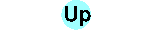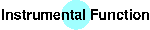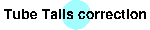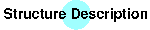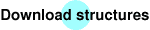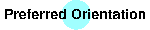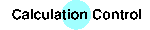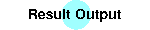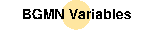# Variables for use with BGMN

The expression interpreter

Variables in the task description SAV file for BGMN
NTHREADS, VAL, VERZERR, STRUC, STRUCOUT, SimpleSTRUCOUT, PDBOUT, RESOUT, FCFOUT, OUTPUT, LIST, RU, UNT, UNTC, DDM, PARAM, LAMBDA, SYNCHROTRON, EPS1, EPS2, EPS3, EPS4, POL, PROTOKOLL, ONLYISO, ITMAX, DIAGRAMM, PLAN, STANDALONEPLAN, GOAL, WMIN, WMAX, CUT, LIMIT2, LIMIT4, LIMIT6, LIMIT8, LIMIT10, ANISOLIMIT, ANISO4LIMIT

Items in the structure description *.str-file
Definition of the lattice
SpacegroupNo, HermannMauguin, GeneralCondition, A, B, C, ALPHA, BETA, GAMMA, UNIT
Anisotropic variables
ANISO, ANISOLIN, ANISOSQR, ANISO4
Scaling factor/prefered orientation
GEWICHT, SPHAR0, SPHAR2, SPHAR4, SPHAR6, SPHAR8, SPHAR10
Real structure
B1, B2, k1, k2, k3, sk, H, h, k, l, zweiTheta, RP
Peak deselection
GeneralCondition
Atomic positions
E=, Wyckoff, x, y, z, TDS, B, betaij, U, Uij
GOAL's, quant analysis
GOAL, GEWICHT
BGMN-specific functions
PHASE, sk, B1, k2, ANISO, GEWICHT(h,k,l), GrainSize, TDS,
RefMult, iref, GEWICHT[i], B1[i], B2[i], k1[i], k2[i], k3[i], DELTAsk, DELTAzweiTheta, LeBail, FPARAM
F, Finv, H
Advanced feature: User calculated structure amplitudes
FMult, F[i], phi[i], Finv[i], phiinv[i]
set, setgitter, cross, diffvec, normvec, skalpro, distance, angle, cpXYZ, T, D, X, Y, Z, WW, WWalt, Straf, Theory, Bondings, BondLevel
Micro absorption correction according to Brindley
my, my[i]
X ray density
density, density[i]

Variables in the task description SAV file for computation of standard profiles
NTHREADS, VERZERR, TubeTails, R, FocusH, FocusW, HSlitR, HSlitW, RoundSlitR, RoundSlitD PColl, PCollA, VSlitR, VSlitH, SamplD, SamplW, SamplH, DeltaOmega, SCollA, SSlitW, SSlitR, SColl, SCollA, DetW, DetH, DetArrayW, MonR, MonH, EPSG, zweiTheta[i], GSUM, TSlitR, TSlitH, FocusS, FocusA, GEOMETRY, WMIN, WMAX, WSTEP, GEQ, D, T, STANDARDPAR, VAL,

## The expression interpreter

BGMN uses a built-in expression interpreter. This interpreter in general uses case-sensitive variable names of unlimited lenght. The number of indices per variable is unlimited. Indexed variables are referred as follows:
```name[i,j,k]
```
Variables may hold numeric values (double format) or strings. In special, a string may hold an expression. Expressions are stored as-is and evaluated every time they are used. Therefore expression evaluation may become recursive: An expression may contain a variable, which refers to a second expression etc. Bracket level is unlimited. A lot of standard functions are predetermined:
abs, sqr, sqrt, sin, cos, tan, asin, acos, atan, log, exp
with one argument. As usual, the argument of the angular functions sin, cos,tan and the result of its inverses (asin, acos, atan) are in radians.
mod, power
with two arguments,
min, max
with variable argument count,
ge, gt, le, lt, eq, ne
This functions accept two or more arguments.
ge, gt, le, lt, eq return 1.0 (TRUE), if the condition holds for each neighboured pair of arguments, starting from the first. If some pair does not hold the condition, 0.0 (FALSE) will be returned immedially, errors in following arguments will not cause this function to cause errors.
ne looks for inequality between any pair (also not neighboured) of arguments. If two arguments are equal, ne returns 0.0 (FALSE). Otherwise, ne returns 1.0 (TRUE).
and, or
with variable argument count. Evaluation of arguments is done until the result is determined (C-like evaluation).
not
with one argument,
ifthenelse
with three arguments. If the first argument is TRUE, then the result of the second expression is returned, otherwise the result of the third. The other argument will cause no error to the result.
select
with variable argument count returns the value of the i+1-th argument depending on the value of the first argument. An error occurs if the value of the first argument is zero or below or above the select parameter number. The other parameters will cause no error if they are undefined or cannot be evaluated as a number. Examples:
```    select(2,1,3,5)
```
return 3,
```    select(1,4,2,7)
```
returns 4.
integral(y,x,xu,xo)
with four or five arguments calculates a numeric integral. Integrand will be y, the integral will be carried out over x between xu and xo. For minmization of the numeric effort, an adaptive 7-point-formula will be used. The precision of the numeric integration may be set as a fifth parameter, default is 1E-13 (which will, for the fast converging 7-point formula, reach a precision near to 1E-15). Example:
```    integral(sin(x),x,0,acos(0))
```
will calculate 1.0.
cintegral(phi,yabs,yphase,x,xu,xo)
with six or seven parameters calculates a complex numeric integral. The absolute value of the integrand will be yabs, its complex phase angle yphase. The integral will be carried out over x between xu and xo. For minmization of the numeric effort, an adaptive 7-point-formula will be used. The precision of the numeric integration may be set as a fifth parameter, default is 1E-13 (which will, for the fast converging 7-point formula, reach a precision near to 1E-15). cintegral returns the absolute value of the integral, its phase angle will be set to the variable given at first position (phi). Example:
```    cintegral(phi,1,90*x/acos(0),x,0,acos(0))
```
will calculate the square root of 2, phi will be set to 45.
ifdef
with variable argument count. Delivers TRUE, if all arguments may be evaluated to a numeric (double) value. In the case one value is undefined (causes an error), ifdef results in an error.
cat
Concatenation of commands, with variable argument count. Arguments may be expressions or assignments. At least one expression must be in the argument list, the rightmost expression determines the return value of the function.
while
looping of commands, with variable argument count. The first argument must be an expression. As long as its value is non-zero and no error occurs, all following arguments are evaluated. Returns the number of loop iterations.
ergibt
with one argument, executes the assignment given as argument. Returns TRUE, if the assignment is succesfull, otherwise FALSE.
gauss, cgauss
are high-level standard functions for solving linear equation systems. Called as
```    gauss(A,x,b,eps,n)
cgauss(Areal,Aimag,xreal,ximag,breal,bimag,eps,n)
```
A,x,b are placeholder for arrays, therefore only non-indexed names are allowed. Both the standard functions solve the inhomogen linear equation system
Ax=b
where A is the matrix A[1,1]...A[n,n], b is the right side b...b[n] and x is the unknown solution x...x[n]. eps is a limit for the pivot element, if any pivot element is smaller than eps the equation leaves unsolved and zero (false) will be returned. If the equation system has been solved and x...x[n] hold the solution, 1 (true) will be returned. `cgauss` is the equivalent for a complex array A, a complex right side b and a complex unknown x.
Please note the special meaning of multiple equation symbols in value assignments to variables:
```a=b+c
```
assigns the string `"b+c"` to the variable named `a`. Every multiple assignment symbol makes one deeper evaluation. e.g.
```d==a
e===a
```
`d` holds the string `"b+c"`. On case `b` as well as `c` may be evaluated to numeric values, the triple assignment `e===a` will set `e` to the numeric value of `b+c`. Otherwise, the assignment `e===a` will cause an error.
```name(argumentcount):expression
```
A positive count of argument defines a fixed value, the absolute value of a negative argument count defines a lower limit of argument count. expression may contain the special symbols
```#1 #2...
```
which are replaced by the evaluated numeric value of the first, second... argument. Every argument is evaluated only once. An errorneous argument causes an error only if it will be used. Example:
```faculty(1):ifthenelse(eq(#1,0),1,#1*faculty(#1-1))
```
or using level 3.3.29 or above:
```faculty(1):cat(n==#1,ret==1,while(n,ret==ret*n,n==n-1),ret)
```
BGMN defines a lot of structure-description specific functions with limited validity. Most of them are only valid in the structure description files. Some are only valid in GOAL's, others only in the description of atomic positions (E=...).

## Variables in the task description SAV file for BGMN

This variable controlls the parallelization of the BGMN code. Up-to-date PCs have multicore processors. For example on a dual core processor you may select
```    NTHREADS=2
```
and most of the time-consuming parts of the BGMN code will be distributed over both the cores. The parallel eficiency of BGMN may be above 90% on Linux or Mac machines with capable processors. You may put the above assignment into the bgmn.cfg file, so it will valid for all BGMN runs.
VAL, VAL...
Files with pattern data. BGMN assumes patterns in free XY[E] format, in general: Leading comments starting with # will be ignored, all following lines may contain
1. angle 2 Theta
2. intensity (cps)
3. (optionally) esd.
If esd is omitted, it will be calculated as the square root of the intensity.

Special file formats are chosen depending from the filename suffix:

• *.val files will be assumed to be in the APX file format of the firm SEIFERT FPM.
• *.rd files with be assumed to be in an old PHILLIPS format. In this case the variable STEPWIDTH may be uses to assign a non-raster stepwidth (STEPWIDTH≠n*0.005)of the pattern data (e.g. PHILLIPS format in conjunction with a scanning PSD from SIEMENS).
• *.raw files will be assumed to be in an old SIEMENS format.
• *.gsa files will be assumed in GSAS format, restricted to equidistant angular steps (both the variants STD and ESD of the GSAS data format).
VERZERR
name of the GEQ-file (output of makegeq). This is the recommended case. If only the GER-file (output of geomet) exists, BGMN interpolates it for every measuring point. If no file is available, you can give an expression as value. This expression may depend from the variable zweiTheta (two Θ). It describes the width parameter of a single squared Lorentzian function, which is used instead of the complicated sum of squared lorentzians. The width parameter refers to Θ, unit radian.
STRUC, STRUC...
Structure description files for the phases present in the specimen.
STRUCOUT, STRUCOUT...
Output of structure descriptions in the same format as the input (*.str).
SimpleSTRUCOUT...
Generates "Simple Structure" output. These files will contain no atomic position data. Instead of, a full list of structure factors depending from h k l (plus, for compatibility, iref) will be generated.

In case you want to convert a LeBail structure refinement into a Simple Structure, you must provide proper values of GEWICHT and density. E.g. while refinement of a 50:50 mixture of an internal standard and a LeBail structure, you should place an assignment of the GEWICHT value as retrieved from the internal standard to the GEWICHT value inside the LeBail structure.

PDBOUT, PDBOUT...
Output of the structures in the *.pdb format (Brookhaven Protein Data Base, e.g. usable for image generation with RasMol)
RESOUT, RESOUT...
Output of the structures in ShelX format.
FCFOUT, FCFOUT...
Output of observed and calculated F's plus phase angle in ShelX format. I have choosed the No. 5 format from the ShelX 97 manual. Cited from those manual:
Write h, k, l, Fo, Fc, and f (phase angle in degree) ... This is indented for input to somestandard macromolecular FFT programs (such as W. Furey's PHASES program), thereby providing a possible route to a graphical display of the electron density.
New in version 2.5.0!
New in Version 2.5.1: All Fs and the phase angles are corrected for dispersion. This means: They really are fourier coefficents of electron density maps. See (newest) ShelX manual.

This output is disabled if the `i`-th phase uses the "user calculated structure amplitudes" feature by defining `FMult` it its `*.str` file.

OUTPUT
*.par-file (peak parameters of all peaks). Used for diagramm generation with SHOW and for peaklist generation with OUTPUT.
LIST
General result output
RU
Number of background parameters. If omitted, BGMN automatic selects a value depending from the 2Θ range and the data accuracy.
UNT
A single file with pattern data. If this parameter is used, its intensities describes a kind of "amorphous background" and are multiplied with the last background parameter and added to the model during refinement.
UNTC
A single file with pattern data, If this parameter is used, its intensities will be added to the model during refinement (no scaling with a refined parameter at all).
DDM
If set to
```    DDM=Y
```
the DDM method as described in

L. A. Solovyov
Full-profile refinement by derivative difference minimization
J. Appl. Cryst. 37 (2004), pp. 743-749
http://www.geocities.com/l_solovyov/ddm.html

will be used. As inherent to this method, the number of background parameters RU is set to zero. The DDM method is indented to cases with complicated background.

PARAM, PARAM...
Global parameters for the fit. Needs a starting value in the kind
```    PARAM[i]=name=startingvalue
```
Possible extensions are
```    PARAM[i]=name=startingvalue_lowerlimit
PARAM[i]=name=startingvalue^upperlimit
```
which may be combined.
LAMBDA
specifies a wavelength file, for example LAMBDA=CU selects the file cu.lam for the wavelength distribution (profile of the K alpha doublet) and cu.ano for the corresponding anomalous dispersion.
SYNCHROTRON
same as LAMBDA, but switches on some specialities for synchrotron radiation. Knows an extended form as
```    SYNCHROTRON=0.079776
```
for specifying an ideal narrow (delta-peak) wavelength distribution at the given wavelength in nanometers. Then, no anomalous dispersion may be specified.
EPS1, EPS2, EPS3, EPS4
describes angular corrections of the measured diagram:
• EPS1 describes the shift of X ray tube and/or detector in radian. Positive values of EPS1 correspond to the tube's resp. detector shift towards the Θ=90° position and therefore to shifting the pattern towards lower angle.
• EPS2 describes the shift of the specimen out of the goniometer axis. Again, the unit is radian. Positive values of EPS2 correspond to:
GEOMETRY=REFLEXION, GEOMETRY=CAPILLARY:
shift of the specimen out of the zero line and orthogonal to this line opposite of the side of tube/detector, which means shift of the pattern towards lower angle. The shift of the specimen is equal to:
R*EPS2/2
GEOMETRY=TRANSMISSION:
shift of the specimen parallel to the zero line in detector direction. The shift of the specimen is equal to R*EPS2/2
• EPS3 corrects for penetration depth. Should only be used with GEOMETRY=REFLEXION, a better way is correction of the profile by penetration depth using the D variable
• EPS4 should only be used without modelling the apparatus function (without having runned geomet/makegeq and without using *.ger/*.geq-files)
POL
used in the polarisation factor. Default POL=1 means no monochromator. Cannot be declared as parameter. Should be in the kind
```    POL=sqr(cos(2 ThetaMonochromator))
```
For Cu Kα radiation and graphite monochromator, use
```    POL=sqr(cos(26.6*pi/180))
pi=2*acos(0)
```
PROTOKOLL
determines, wether a iteration protocol is given or not.
Y/y/J/j: A protocol is printed.
N/n: A protocol is not printed.
Default value is N.
ONLYISO
if this value is set to Y/y/J/j, only a fast isotropic computation is done. All anisotropies and spherical harmonics are ignored. A numeric value controls the exit from iteration. In the case of Y, 1.0E-4 is assumed. Default value is N.
ITMAX
Maximum stepcount while iteration. BGMN uses the 10 fold of the parameter count. In critical cases (e.g. structure solution) this limit should be enlarged.
DIAGRAMM
In every iteration step, a file containing a line per measuring point is written. Each line contains

2Θ Imess Icalc Ibackground

, followed by a list of intensities, each for every phase involved in the refinement. This is for graphical observation of the refinement progress (Diagram window in BGMNwin)
PLAN
A simple list, each line contains angle, count and measuring time of a futural measurement. Output of (optional) Optimal Experimental Design.
STANDALONEPLAN
Logical switch. Decides, if the Designed Measurement has to be evaluated together with the unplanned pre-measurement (STANDALONEPLAN=N) or standalone (STANDALONEPLAN=Y). For a single task, I would prefer N, which is the default. However, in the environment of a routine analysis in a factory, which has to been done cyclically every few minutes (specimen does not change very largely), STANDALONEPLAN=Y becomes significant.
GOAL, GOAL...
global goal of the experiment. Possible values are:
```    GOAL[i]=expression
GOAL[i]=expression=value
GOAL[i]=expression=value+-σ
```
The second line is written in the output SAV file. It contains the result. The third line ist written in the LIST file, supporting a fitted value and an error (1σ) for the expression. If one uses the third line in the SAV-file, Optimal Experimental Design is done and the σ value should be reached after a planned measurement an fit of both measurements at once.
WMIN, WMAX
Angular limits for selection of measuring values, unit degree in 2Θ.
CUT, CUT...
Angular limits for deselection of measuring values. Format:
```    CUT[i]=wmin:wmax
```
, units are again degree in 2Θ
LIMIT2, LIMIT4, LIMIT6, LIMIT8, LIMIT10, ANISOLIMIT, ANISO4LIMIT
These variables controls the automatic reduction of degree of spherical harmonics and/or anisotropies. After isotropic calculation, the value of the variable GEWICHT must exceed the LIMITx-fold of its error (1σ). Otherwise, the degree of spherical harmonics is reduced. ANISOLIMIT, ANISO4LIMIT works in the same kind for any variable declared as ANISO or ANISO4. ANISO4 is reduced to ANISOSQR. If not given (which should be mostly the case), BGMN chooses an advanced value depending from the Laue Group of the phase.

## Items in the structure description *.str-file

### Definition of the lattice

Beside there are other ways for defining a lattice with BGMN, one should use the spacegrp.dat file. Therefore, give at least one of the items
```HermannMauguin=...
SpacegroupNo=...
```
in the structure description file. In addition, one may set
```GeneralCondition=...
```
as a bool switch for the selection of the reflection (depending on h k l) conditions as given in the International Tables. Zero (false) will omit the reflection, non-zero (true) activates the reflection. And give the non-trivial lattice constants, e.g.
```A=... C=...
```
for tetragonal or hexagonal lattice. The full set of lattice constants (triclinic) must be named
```A=... B=... C=... ALPHA=... BETA=... GAMMA=...
```
In normally, this lattice constants should be parametrized and boundaries should be used, e.g.
```PARAM=A=0.314_0.312^0.316
```
Attention! Units of measure are nanometers, or you must use the key
```UNIT=ANGSTROEM
```
in this structure description file!

### Anisotropic variables

Each variable may be parametrized. As an extension, each variable may be parametrized anisotropic by using the key's
```name=ANISOLIN
name=ANISOSQR
```
There are some special keys for backward comptibility:
B1=ANISO stands for B1=ANISOLIN
k2=ANISO stands for k2=ANISOSQR
GEWICHT=ANISO stands for GEWICHT=ANISOSQR
ANISO4 is recommended in the case of complicated micro strain, use k2=ANISO4. This means a fully symmetric product of hkl with a positive definite tensor of forth rank. See:
• P. Thompson et al.,J. Less Common Met. 129 (1987) 105
• J. Rodriguez-Carvajal et al., J. Phys. Condens. Matter 3 (1991) 3215
• Both cited corresponding to an oral presentation of P.W. Stephens on EPDIC 5, Parma, Italy
Each of this key's may be completed by an upper limit, e.g.
```B1=ANISO^0.02
```
This boundary is valid only in the first steps of computation. In this steps isotropic replacements for such variables are used. Therefore, the limit is only a hint for the iteration procedure.

The symmetriy of the anisotropic values is automatically corrected to the lattice symmetry.

### Scaling factor/prefered orientation

The commonly used scaling factor is predefined by the variable
```GEWICHT
```
Of course, one may define this variable as anisotropic. For strong and multiple prefered orientation there are possible spherical harmonics. The keywords therefore are:
```GEWICHT=SPHAR0/SPHAR2/SPHAR4/SPHAR6/SPHAR8/SPHAR10
```
Only the even coefficients up to the given order are used. This means 6/15/28/45/66 independent parameters in triclinic lattices. Of course, the symmetry of the spherical harmonics is atomatically corrected to the lattice symmetry. Plus, BGMN guarantees these spherical harmonics to be positive definite. For details see:
J. Bergmann, T. Monecke, R. Kleeberg
Alternative algorithm for the correction of preferred orientation in Rietveld analysis
J. Appl. Cryst. 34 (2001) pp. 16-19

The effective count of parameters is reduced, thereby, for all non-triclinic lattices. SPHAR0 corresponds to an isotropic value.
GEWICHT is corrected with the usual factors for weight content analysis. This includes in special the value as predefined in the variable density, which is defined from the sum over all FMult atomic settings in case FMult is set. In case FMult is not set, density defaults to that of the first subphase. In General, SPHARx starts with the isotropic value zero. But if no measuring data are given (only theoretic computation), the value 1 is assumed.
If both GEWICHT=SPHARx and GEWICHT=ANSIO are active:

• GEWICHT=ANISO dominates over GEWICHT=SPHAR0.
• GEWICHT=SPHARx (x>0) dominates over GEWICHT=ANISO.
• By defining GEWICHT=ANISO and GEWICHT=SPHARx (x>0) one gets the extended switching SPHAR6->SPHAR4->SPHAR2->ANISO->ISOTROPIC, which makes senso in complicated cases.

### Real structure

Having solved the problem of wavelength and apparatus influence into the profil shape, BGMN now must handle the specimens influence to this shape. This is normally done by three variables, two of them may be declared anisotropic:
B1
stands for a lorentzian broadening caused by size effects. It should be parametrized. Units of measure is the inverse of that used for the lattice constants, in common 1/nm. You are encouraged to declare it anisotropic using the key
```    B1=ANISO
```
if the specimens crystals are of anisotropic shape.
B2
Is the square of the broadening of a squared lorentzian, which is more gaussian-like. Using the square of the broadening solves a convergence problem, which will occur for B2=0 by handling the squared lorentzian with itself. In usual, you should not use this variable direct. BGMN predetermines the formula
```    B2=k1*sqr(b1)+k2*sqr(1/d)+k3*sqr(cost*max(0.0,cot(twoTheta)))
```
k1 describes a gaussian-like part of the size effects. It should be parametrized in the way
```    PARAM=k1=starting_value_0^1
```
Values greater 1 leads to negative contents of smallest particles in the particle size distribution. The greater the value of k2, the narrower is the particle size distribution.

k2 may describe the micro strain effect. It is the quare of the usual micro strain. You may describe it anisotropic by using

```    k2=ANISO
```
or
```    k2=ANISO4
```
But k2=ANISO should be restricted to very special cases, for which you have any hints for the reallity of anisotropic strain.

You should never use k3, except in case you don't use any predetermined specimens function's (which are generated by running geomet/makegeq and sored in *.ger/*.geq files). In such cases, k3 describes a broadening of the line for small angles. Asymmetry is never described, in this way!

You may assign own expressions to B1 and/or B2. There are some predefined variables for doing so:
sk
holds the 1/d-value of the reflection, unit 1/nanometer (or 1/Å in case you use UNIT=ANGSTROEM for this structure file).
h, k, l
hold the Miller indices of the reflection. Attention: In general, reflections are multiple. For each reflection only one triple of Miller indices is used for computation, and intensity is multiplied with the multiplicity!
zweiTheta
holds the 2Θ value of the reflection.
H
holds the multiplicity of the reflection.
The kind of the profile model is controlled by the variable RP, also.
RP=2
means ideal lines, no specimen influence on line shapes.
RP=3
means usage of Lorentzian-broadening B1 only.
RP=4
means usage of both Lorentzian B1 and squared Lorentzian broadening B2. This is the default value.

### Peak deselection

The term as assigned to `GeneralCondition` should depend from h k l. Enables the deselection of peaks from the pattern. Example:
```GeneralCondition=and(eq(h,0),eq(k,0),eq(abs(l),2)
```
will select the 002 reflection only, for hexagonal lattice. Of course, such a selection must select/deselect all equivalent reflections in an equal manner.

### Atomic positions

In general, the key for starting an atomic position is the position's occupation definition, e.g.
```E=C
E=(NA(p),K(1-p))
E=(NA(p),K)
```
The last two lines are equivalent. All non-weighted elements are used to complete the position's total occupation probability to one. Ionic charges may be specified:
```E=C+4
```
For a list of all defined ionic charges, see file `afaparm.dat`. For neutron data, an isotopic number may be specified:
```E=C12
```
Both the ionic and isotopic selectors may be combined:
```E=C12+4
```

```E(a,b,c...)=
```
is a special definition for the case `FMult>0` or `RefMult>1`. In case `FMult` is set, it defines the contributions of the atomic position to the different `F[i]` etc. Otherwise, it defines the contributions of the atomic position to the different subphases `1...RefMult`.

The next is the Wyckoff symbol in accordance to the spacegrp.dat file, e.g.

```Wyckoff=c
```
The next are the x, y, z values as demanded by the Wyckoff symbol:
```x=0.312 z=0.574
```
For structure refinement, these values may be parametrized:
```PARAM=x=0.312_0.31^0.315
```
The Debye-Waller-Factor is given by the variable TDS. It may be declared as anisotropic:
```TDS=ANISO
```
The isotropic TDS value is equal to the common used B, except for the unit of measure (default nm**2, otherwise use UNIT=ANGSTROEM). The anisotropic TDS values given in the LIST file are dimensionless and are equivalent to the βij values. The Uij values also are given in the LIST file, Uij values are given in the used unit of measure. If you have given only an isotropic U, please use the equation

TDS=8π2U

If no TDS is defined, a phase-specific of measurement-global expression for TDS is used. Otherwise, TDS is assumed to be zero.

### GOAL's, quant analysis

A special feature of BGMN are GOAL's. From above, you know them as global GOAL's. You can, of course, use them in phase-specific or position-specific context. Therefore you must omit the index, e.g.
```GOAL=A/C
```
for determining the lattice constants ratio A/C. A special feature for non-global GOAL's are the so-called auxiliary goals:
```goal:name=expression
```
In opposite to normal assignments, the right side of the auxiliary goal's assignment is evaluated to a numeric (double) value. Furthermore you can use GOAL-time specific functions in auxiliary goals.

Quant analysis is supported by the GOAL's. Therefore, in each phase's structure description file use the auxiliary GOAL

```GOAL:phasei=GEWICHT
```
In the case of three phases, use the three GOAL's
```GOAL=phase1/(phase1+phase2+phase3)
GOAL=phase2/(phase1+phase2+phase3)
GOAL=phase3/(phase1+phase2+phase3)
```
in the SAV file. The values for GEWICHT are corrected for the phase's X ray density (that of the 1st Subphase in case RefMult>1). So they can used for quant analysis, directly.

### BGMN-specific functions

PHASE
with one argument serves TRUE, if the string given as argument is identic to the phase identificator given for the phase.
sk
uses three arguments: A set of Miller indices. It serves the 1/d value for the given reflection. Usable only in GOALs
B1
uses the Miller indices as three arguments. Serves the B1 value of this reflection. Usable only in GOALs.
k2
uses the Miller indices as three arguments. Serves the k2 value of this reflection. Usable only in GOALs.
ANISO
First parameter is a variable declared as ANISO, followed by three Miller indices. Serves the value of this variable in the given direction. Usable only in GOALs.
GEWICHT
uses the Miller indices as three arguments. Serves the value of GEWICHT for this direction. Usable only in GOALs. As a special feature, the mean value of GEWICHT is set to the variable GEWICHT at GOAL time. So you can get the value of the prefered orientation's correction for a given direction simply by writing
```    GOAL=GEWICHT(h,k,l)/GEWICHT
```
GrainSize
uses the Miller indices as three arguments. Serves the value of a mean grain diameter in the given direction, which is influenced by B1 and k1. Usable only in GOALs.
TDS
uses the Miller indices as three arguments, Serves the value of the Debye-Waller-factor for the given direction. May be used in a position description and only in GOALs. If you defines a global TDS, you may use this GOAL function outside a position description, too.

As an advanced feature, subphase definition may be used for complicated real structures description. Therefore, you must assign a value greater than one to the variable RefMult. Subphases share:
• the values of prefered orientation hold in the variable GEWICHT (which is automatic set in dependence from the Miller indices, in the case of GEWICHT=ANISO/GEWICHT=SPHARx)
• the lattice constants and therefore the variables sk/zweiTheta, which are automatic set, too.
But you can influence this values subphase-specific as described below. The number of the actual computed suphase is set to the variable iref=1,2,... The scaling factors of the subphases may be set by writing:
```GEWICHT[i]=expression
```
If not defined, BGMN uses the variable GEWICHT. You can use the value of the variable GEWICHT as well as some new parameters in the above expression. So you can define arbitrary subphase's scaling factors. You can define subphase-specific values of the real-structure variables

B1, B1...
B2, B2...
k1, k1...
k2, k2...
k3, k3...

and/or you can use the variable

`iref`
in the expressions for non-indexed real structure variables. You can assign arbitrary values to the variable
`DELTAsk`
and/or
`DELTAzweiTheta`
and/or these variables indexed with the subphase's number. Their values are used for position correction of the subphase's peaks.

Setting `LeBail=i` to a value between `1...RefMult` will cause to omit calculation of `F`, `GEWICHT` or `GEWICHT[i]` for that subphase. Instead of, all peak intensities of that subphase will be refined independently (LeBail-fit).

### Advanced feature: Structure amplitudes etc

As an advanced feature, BGMN serves both the absolute values of the structure amplitudes of the reflection {h,k,l} resp. the inverse reflection {-h,-k,-l} by the variables `F` resp. `Finv`. In addition, the multiplicity of the reflection is served by the variable `H`.

In case of combined refinement, all peak intensities are refined independently. That means: Any individual (hkl) triplet gets as many intensity parameters as devices are defined. That holds for `RefMult`>1 too. This behaviour may be changed by setting

```FPARAM=Y
```
(or "Yes" or "yes"). In such case, the structure factors `F` of all occuring (hkl) become parameters. Of course, `GEWICHT` and `density` have to be defined for intensity calculations.

### Advanced feature: User calculated structure amplitudes

One may even set
```FMult
```
to a positive integer. Using `FMult`, BGMN will provide
```F[i]
```
and
```phi[i]
```
for the absolute value resp. phase angle (in degree 0...360) of the complex structure amplitudes as defined in `E(a,b,c)=`. >From that, one may do arbitrary operations and at the end set the structural amplitude `F` of the whole phase, which will then used for intensity calculation.
The values for the inverse reflection are set to `Finv[i]` and `phiinv[i]`.

In case both `Fmult` and `RefMult` are set, one may use `iref` while calculating `F`. Thereby, different `F` values for different subphases may result.

BGMN provides a function `F(phi...)` for dynamic structure factor calculation. Atomic positions as defined in part 2 of the structure file are evaluated prior to all peak parameter calculations. Instead of, `F(phi...` calculates the structure factor for the atomic positions defined within F(phi...) each time it will be called. It returns the absolute value of the complex structure factor, its pahse angle will be set to the variable given at first position (phi). `F(phi...)` may, for example, usde within `cintegral` for evaluation of arbitrary atomic position distributions. Example:

```FMult=1
F=cat(fmittelbetrag==cintegral(fi,F(psi,
D2(r1,r2,rot,EOZ1,eoz1,EOZ2,eoz2,EOZ3,eoz3,EOZ4,eoz4,EOZ5,eoz5,EOZ6,eoz6),
E=NA+1(pCA),Wyckoff=c,XYZ(ECA),TDS==tdsint,
E=O-2(pOZ),Wyckoff=c,XYZ(eoz1),TDS==tdsH2O,
E=O-2(pOZ),Wyckoff=c,XYZ(eoz2),TDS==tdsH2O,
E=O-2(pOZ),Wyckoff=c,XYZ(eoz3),TDS==tdsH2O,
E=O-2(pOZ),Wyckoff=c,XYZ(eoz4),TDS==tdsH2O,
E=O-2(pOZ),Wyckoff=c,XYZ(eoz5),TDS==tdsH2O,
E=O-2(pOZ),Wyckoff=c,XYZ(eoz6),TDS==tdsH2O),
psi,rot,0,120)/120,
sqrt(sqr(F*cos(pi*phi/180)+fmittelbetrag*cos(pi*fi/180))+
sqr(F*sin(pi*phi/180)+fmittelbetrag*sin(pi*fi/180))))
```
will calculate the strucure factor including a rotating octahedron.

As a special feature for structure refinement, you can define a molecule or parts of it in cartesian or atomic (fractional) co-ordinates. In following you can shift and rotate the molecule as a whole or only parts of it (changing bonding lengths, bonding angles or torsion angles in dependence from some refined parameters). As a very advanced feature, you can define some penalty conditions similar to those used in molecular dynamics to avoid molecules from overlapping.

Therefore BGMN uses some special functions:

set
uses 4 parameters. The first is a position descriptor, e.g. C1 for the first carbon atom. The following three are the cartesian co-ordinates of this atom in the molecule. They are assigned to the indexed variables
`C1 C1 C1`
according to the example (in further referred as a vector).
setgitter
makes the same task, except that it reads atomic (fractional) co-ordinates. An error occurs, if this function is used before full definition of the lattice constants.
cross
Arguments must be 4 vectors. The first 3 must be defined. Computes the cross product between two connection vectors: The vector between the first and the second position and the vector between the second and the third position. The result equals the vector between the second and the fourth position (the fourth position is set in this kind).
diffvec
Needs three vectors as arguments. Sets the third vector to the difference of the second minus the first.
normvec
Arguments are two vectors. Calculates the second vector as the unit vector in the direction of the first.
skalpro
Needs two vector descriptors. Calculates the scalar product between both and returns it as the function's result.
distance
Needs two vectors as argument. Calculates the distance between both vectors (the atomic distance).
angle
Needs 2, 3 or 4 vectors as arguments.
• For 2 arguments it returns the angle between both vectors.
• For 3 arguments, it returns the bonding angle between the 3 positions.
• For 4 arguments, it returns the torsion angle between the 4 positions.
The result is served in degree!
cpXYZ
with even parameter count: After having defined the molecule with `set(a,x,y,z)` (or `setgitter(a,x,y,z)`), `cpXYZ(a,b...)` generates copies with identic co-ordinates. Within each pair of parameters, the co-ordinates of the left one are copied to the right-one. The right-one parameter may be a comma-separated list in brackets:
```cpXYZ(a,(b,c,d),e,f)
```
By this way, multiple copies are generated from one source.
T
Needs at least 7 parameters. Use it in the kind:
```    T(x,y,z,alpha,beta,gamma,E1,...)
```
Defines an arbitrary unitary transformation of the positions E1,... At first, all positions are rotated for the three euler angles alpha,beta,gamma. At second, all positions are shifted for the values x,y,z. alpha,beta,gamma must be given in degree!
T2
Needs at least 8 parameters, parameter count must be even.. Quite similar to T, but instead of in-place postion operations it uses in/out position pairs. So you may omit an extra call to cpXYZ:
```    T2(x,y,z,alpha,beta,gamma,E,e)
```
is an abbreviation of
```    cpXYZ(E,e)
T(x,y,z,alpha,beta,gamma,e)
```
D
Needs at least 4 parameters. Use in in the kind:
```    D(A,B,chi,E1,...)
```
Defines a rotation of the positions E1,... around the axis A-B for the angle chi. chi must be given in degree!
D2
Needs at least 5 parameters, parameter count must be odd. Quite similar to D, but instead of in-place postion operations it uses in/out position pairs. So you may omit an extra call to cpXYZ:
```    D2(A,B,chi,E,e)
```
is an abbreviation of
```    cpXYZ(E,e)
T(A,B,chi,e)
```
X, Y, Z
Need each one argument: a position descriptor. Returns the (fractional) atomic co-ordinates of this descriptor. Must be used in definition of any atomic position after
```    E=...
```
for defining the values of x,y,z in accordance with the Wyckoff symbol. E.g.
```    x=X(C1) y=Y(C1) z=Z(C1)
```
XYZ
needs one argument: a position descriptor. Usage:
```    XYZ(C1)
```
is simply an abbreviation for
```    x=X(C1) y=Y(C1) z=Z(C1)
```
WW
Needs at least 5 arguments. It defines an interaction energy in accordance to common used values in molecular dynamics. Use it in the way:
```    WW(a,m,b,n,(A1,A2,...))
WW(a,m,b,n,(A1,A2,...),(B1,B2,...))
```
The interaction energy in common is given as a*rm+b*rn, where r is the distance between both atoms. The results for all significant atomic pairs (also in neighbour elementary cells etc.) are added and multiplied with the value of the variable Theory (default: zero). This value then is added to the minimized value during Rietveld's fit. The first line means all combinations of one list (e.g. all interactions between carbon atoms of a molecule). The second line means all combinations between two lists of atoms (e.g. all interactions between all carbon and all nitrogen atoms of a molecule).

As a special feature, you can define an occupation probability of the position A (usable for multiple conformations):

```    WW(a,m,b,n,(A1,A2(p),...),(B1(q),...))
```
WWalt
Needs one additional parameter to WW. This last parameter is an interaction probability. You need it for correlated occupation probabilities of two atomic positions. In WW, the interaction probability is computed as the product of the two occupation probabilities.
Straf
Needs one argument: An expression. The square of this expression is used as an energy term. So you can define any arbitrary penalty function.
As an additional feature, you can define assignments
```Bondings=E1,E2,...
```
as many as you want. They are controlled by the variable BondLevel (default value 1). This means: Every atom didn't interact (in WW/WWalt) with itself (BondLevel=0), the next bonded atoms (the atoms must be neighbours in any Bondings list, case BondLevel=1), the next next bonded atoms (BondLevel=2) and so on.

### Micro absorption correction according to Brindley

For each phase, there is supported the variable `my`, which holds the linear attenuation coefficient of the phase.

In the case `FMult` is set, `my` holds the sum of the linear attenuation coefficients of all `my[i]`. Otherwise, `my` defaults to `my`. Using `my[i]`, one may compute total my values differing from the above defaults. For a simple Brindley correction (identic grain size for every phase) please use the auxiliary GOAL

```GOAL:phasex=GEWICHT*exp(my*d*3/4)
```
where d holds the mean grain diameter in microns. This is valid since my is given in the unusual unit µm-1

In cases you use other wavelengths than the usual (Co, Cr, Cu, Fe) for which no file *.mdr exist, `my` will be set to zero. This is especially the case when using `SYNCHROTRON=` or `LAMDA=` with an numeric value in the *.sav file for this BGMN run.

```my[i]
```
holds the linear absorption coefficient as caused by all atomic settings contibuting to `F[i]` (in case `FMult` is set). Otherwise, it holds the linear absorption coefficient of the ith subphase, which may be of interest in case of setting `RefMult`.

### X ray density of the phase

The variable
```density
```
holds the sum of the X ray densities density[i] (in case `FMult` is defined), otherwise the X ray density of the 1st sub phase (in case `RefMult` is defined).
```density[i]
```
holds the X ray density as caused from all atomic settings contibuting to `F[i]` (in case `FMult` is set). Otherwise, it holds the X ray density of the ith subphase. From that values, one may compute a user-defined value of density.

## Variables in des task description SAV file for computation of standard profiles

As no other said, all lengths are in millimeters an all angles are in degree.
Number of threads as used for calculation in parallel. Should less or equal the number of CPUs in your PC. As of level 5.0.7, all three geometry programs (GEOMET, MAKEGEQ, VERZERR) are parallelized, altough with quite different efficiency:
GEOMET
will use multiple threads for increased precision: There will be additional angular positions in the generated *.ger file. Parallel efficiency is quite good.
MAKEGEQ
will even use a smaller step size, except for capillary geometry where MAKEGEQ is much slower. Parallel efficiency is far from being 100%.
VERZERR
will speed up, but parallel efficiency is even far from being 100%.
You may put assignments to NTHREADS into the geomet.cfg/makegeq.cfg/verzerr.cfg files and they will valid for all runs of the respective programs.
VERZERR
Name of the GER-file (output of GEOMET and VERZERR) and (if no variable GEQ is defined) the output GEQ-file of MAKEGEQ.
TubeTails
File with pattern data for Tube Tails correction. Implementation restriction: This file may contain only one scan of equidistant data. Possible file formats are identic to the VAL[i] entry.
R
FocusH FocusW
Axial (FocusH) and equatorial (FocusW) dimension of the X-ray tube's focus. FocusW means the optical focus width. Usually, the thermal focus dimensions are printed on the X-ray tube. The optical equatorial focus dimension is reduced by the take-off angle of X-rays from the anode surface (usually 6°). In following, the optical equatorial focus dimension equals the 10th part of the thermal one.
HSlitR, HSlitW
Radius (distance from device axis) and width of the equatorial divergence slit. May depend from the variable zweiTheta, which means variable divergence slit. In this case GSUM=Y should be used.
RoundSlitR, RoundSlitD
Radius (distance from device axis) and diameter of a round slit (plate with a circular hole) in the primary beam.
PColl
Divergency angle of the primary soller slit, e.g. 0.5/25 (unit is radian). Default is no collimator.
PCollA
Out-of-plane-angle of primary soller slit.
VSlitR, VSlitH
Radius (distance from device axis) and width of the axial divergence slit.
SamplD
Diameter of a round specimen. Default value is infinite (sufficient large). Using a small value, you should give GSUM=Y.
DeltaOmega
Ω twist of the specimen, valid only for `GEOMTRY=REFLEXION`. Changes profile shapes plus intensities (GEOMET) and changes effective penetration depths depending from `zweiTheta` (MAKEGEQ). May depend from `zweiTheta`. Therefore, may be used for simulating grazing incidence, too.
AirScat
`AirScat=` may be set for defining an air scatter blocker (a metal plate perpendicular to the sample surface, the goniometer axis within the plane of the plate). `AirScat` should be set to the height of the blocker above sample surface. Valid only for `GEOMETRY=REFLEXION`.
SamplW, SamplH
Length and equatorial dimension of a rectangular, not rotated specimen. Default values are infinite (sufficient large).
SSlitW, SSlitR
Radius (distance from device axis) and width of the anti-scatter slit. May depend from the variable zweiTheta, which means variable anti-scatter slit. If used in conjunction with TubeTails, you should use the switch `GSUM=Y`.
SColl
Divergency angle of the secondary soller slit.
SCollA
Out-of-plane-angle of secondary soller slit.
DetW, DetH
Equatorial (DetW) and axial (DetH) dimension of the receiving slit. DetW may depend on Bragg angle zweiTheta in cases of variable receiving slit, as described for variable divergence slits.
DetArrayW
Total equatorial dimension (in mm) of a 1D array of detectors such as Vantec-1, Lynxeye (Bruker) or X'Celerator (Panalytical). In such cases, DetW should be assigned the equatorial dimension of a single detection unit and DetH the axial dimension of the detection units.
MonR, MonH
Radius of a secondary monochromator (beam path length from device axis). It's axial dimension is smaller than those of the detection slit, in most cases. Therefore give MonR and use the monochromator crystal's axial dimension as MonH.
EPSG
Computational accuracy. Default value: 0.7 per cent.
zweiTheta zweiTheta...
2Θ values, for which geomet computes profile shapes.
GSUM
Logical switch. Values J/j/Y/y means: A variable GSUM is computed and written to each profile in the GER file. GSUM holds the relative intensity of each profile. Use it for small specimens, variable divergence slits etc.
TSlitR, TSlitH
The usage of an additional axial slit near the X-ray tube is supported. These variables describe it's distance from device axis and axial dimension. Optional.
FocusS, FocusA
These variables describe a axial shift and an rotation angle (around the line tube-specimen) of the X-ray focus. Optional.
GEOMETRY
Measuring geometry. Default value is REFLEXION. The value TRANSMISSION describes a planar, thin transmission specimen. The value CAPILLARY describes a thin, wire-like specimen in the goniometer axis. In the case CAPILLARY, SamplD or SamplH describes the length (heigth) of the specimen, and T desribes the diameter of the wire (capillary).
WMIN, WMAX
Angular range for the computation done by makegeq. Default range: 20°...140°
WSTEP
Stepwidth of makegeq. Default value is 0.01 degree, higher values are recommended. May depend from zweiTheta.
GEQ
Output file of makegeq. Default value: The value of the variable VERZERR, but with the extension geq.
D
Reciprocal value of the linear absorption's coefficient of the specimen. Default value: zero (no correction of the profile shape for penetration depth)
T
Thickness of specimen (GEOMETRY=REFLEXION/TRANSMISSION) or capillary/wire diameter (GEOMETRY=CAPILLARY). Default value: Infinity (sufficient thick) in the case REFLEXION, zero (thin specimen or capillary) in the cases TRANSMISSION/CAPILLARY.
STANDARDPAR
Name of the `*.par` file containing only the selected reference lines of the standard specimen. Used for learnt profiles.
VAL[x]
Enumeration of the measured scans, which will be accumulated by the VERZERR program. Used for learnt profiles.

Attention: Take care to unify all multiple reflectios. Unequal crystallographic directions giving identic 1/d should be unified to one line in this file.